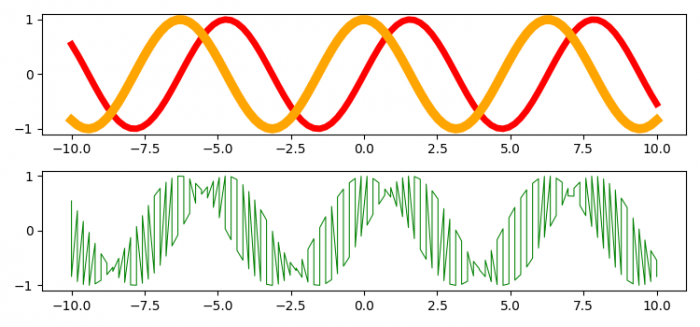# How to merge two existing Matplotlib plots into one plot?

To merge two existing matplotlib plots into one plot, we can take the following steps −

• Set the figure size and adjust the padding between and around the subplots.
• Create x, y1 and y2 data points using numpy.
• Plot (x, y1) and (x, y2) points using plot() method.
• Get the xy data points of the current axes.
• Use argsort() to return the indices that would sort an array.
• Append x and y data points of each plot.
• Plot X and Y data points at the 2nd index subplot.
• To display the figure, use show() method.

## Example

import numpy as np
import matplotlib.pyplot as plt

plt.rcParams["figure.figsize"] = [7.50, 3.50]
plt.rcParams["figure.autolayout"] = True

x = np.linspace(-10, 10, 100)
y1 = np.sin(x)
y2 = np.cos(x)

plt.subplot(211)
plt.plot(x, y1, color='red', lw=5)
plt.plot(x, y2, color='orange', lw=7)

X, Y = [], []

for lines in plt.gca().get_lines():
for x, y in lines.get_xydata():
X.append(x)
Y.append(y)

idx = np.argsort(X)
X = np.array(X)[idx]
Y = np.array(Y)[idx]

plt.subplot(212)

plt.plot(X, Y, color='green', lw=0.75)

plt.show()

## Output